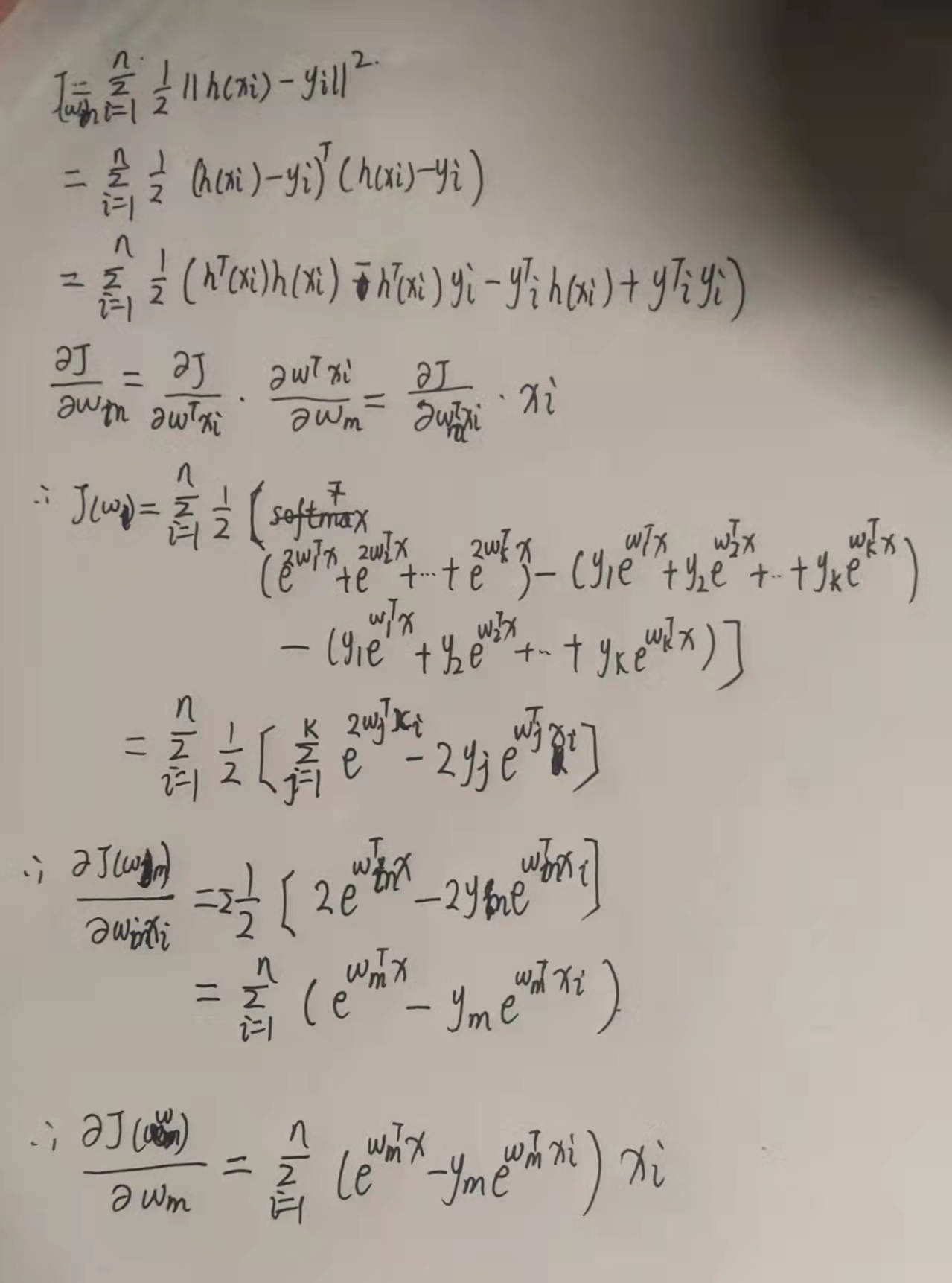# 逻辑回归（Logistic Regression)详解

## （1）逻辑回归所处理的数据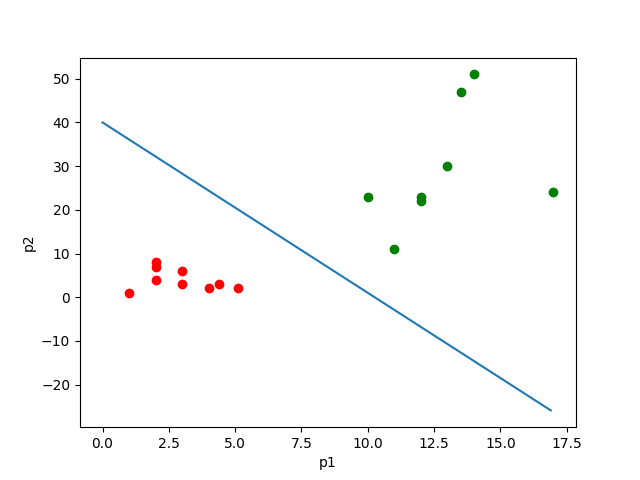如上图所示，其中点的个数是样本个数，两种颜色代表两种指标。这个直线可以看成经这些样本训练后得出的划分样本的直线。那么对于之后的样本的p1与p2的值，就可以根据这条直线来判断它属于哪一类了。

w写成,写成就可以写成,则：

## （3）求解参数。

（1）极大似然估计。

.

（2）损失函数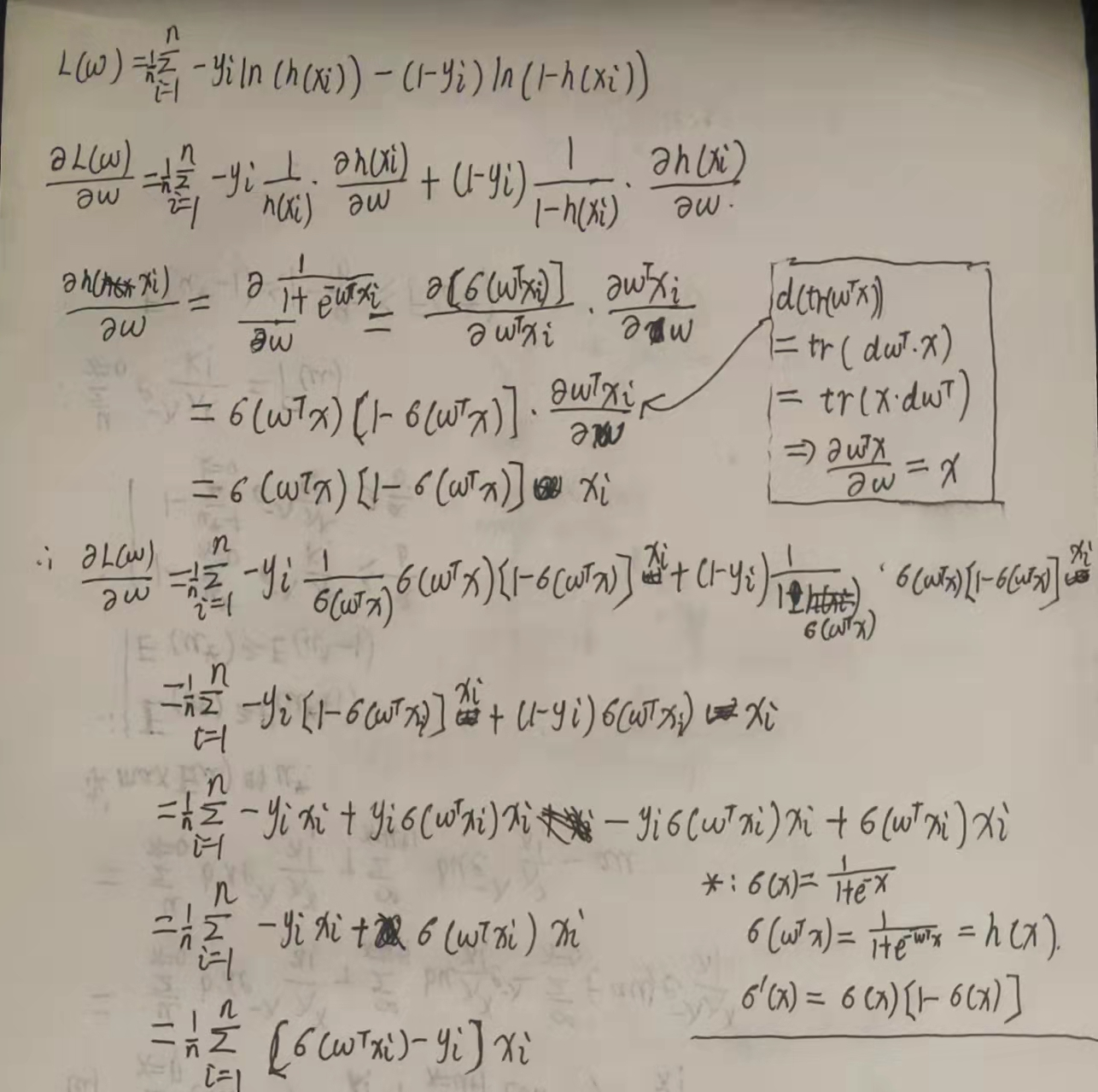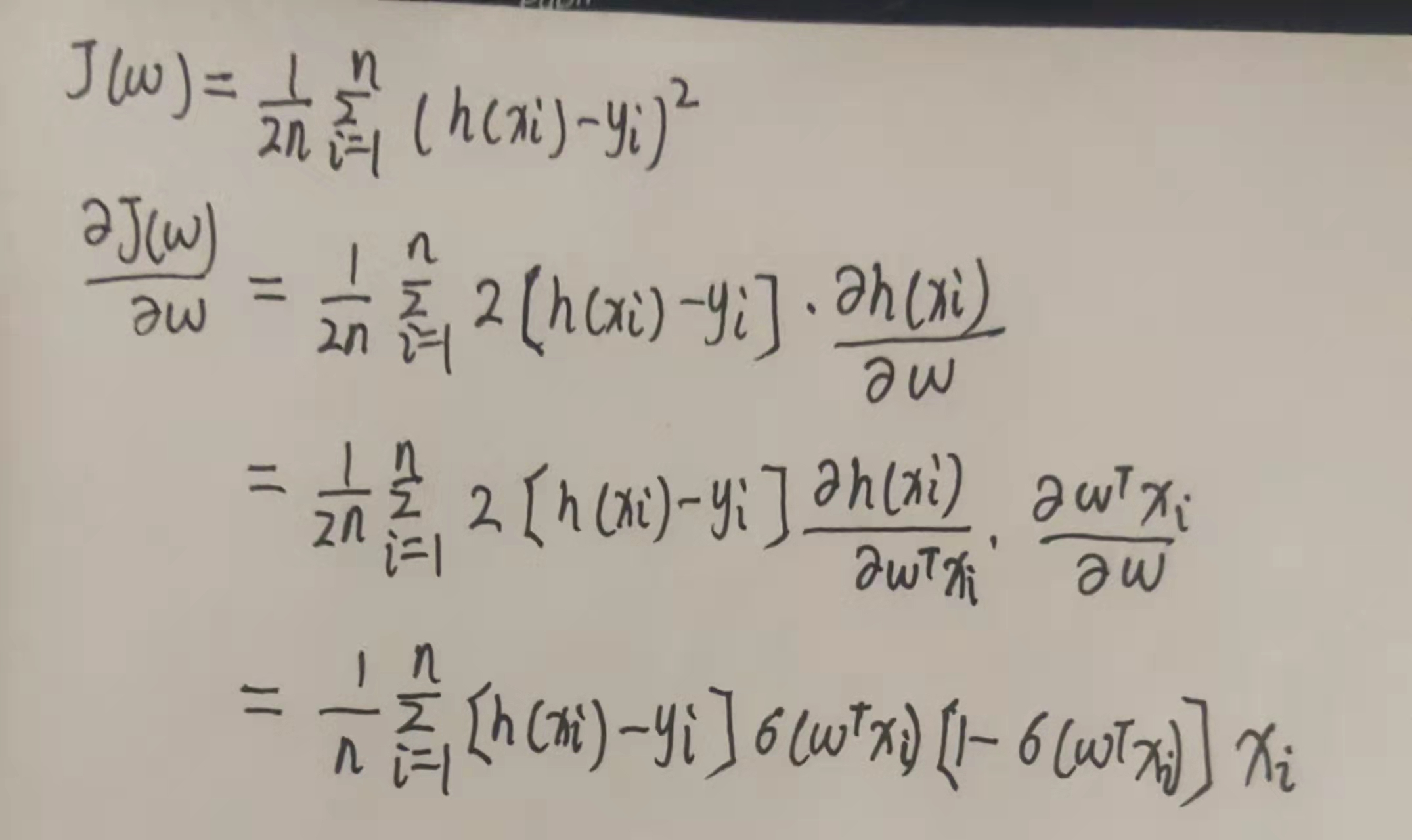## （3）算法实现。

import pandas as pd
df=pd.DataFrame(data)
print(df.columns，df.shape)

import pandas as pd
import matplotlib.pyplot as plt
df=pd.DataFrame(data)
color=[]
for i in df['Class'][0:3349]:
if i=='SEKER':
color.append('red')
else:
color.append('blue')
plt.scatter(df['MajorAxisLength'][0:3349],df['MinorAxisLength'][0:3349],color=color)
plt.xlabel('MajorAxisLength')
plt.ylabel('MinorAxisLength')
plt.show()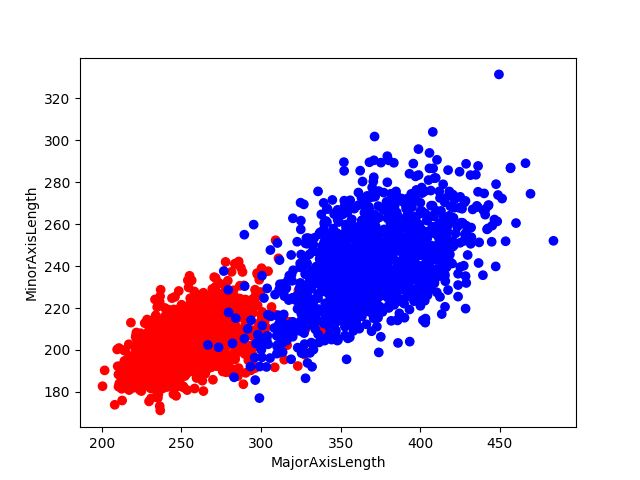可见，该数据是可以用一条直线分成两个部分的。

import numpy as np
import pandas as pd
df=pd.DataFrame(data)
label=[]
for i in df['Class'][0:3349]:
if i=='SEKER':
label.append(0)
else:
label.append(1)
x1=df['MajorAxisLength'][0:3349]
x2=df['MinorAxisLength'][0:3349]
train_data=list(zip(x1,x2,label))
class Logistic_Regression:
def __init__(self,traindata,alpha=0.001,circle=1000,batchlength=40):
self.traindata=traindata #训练数据集
self.alpha=alpha #学习率
self.circle=circle #学习次数
self.batchlength=batchlength #把3349个数据分成多个部分，每个部分有batchlength个数据
self.w=np.random.normal(size=(3,1)) #随机初始化参数w
def data_process(self):
'''做随机梯度下降，打乱数据顺序，并把所有数据分成若干个batch'''
np.random.shuffle(self.traindata)
data=[self.traindata[i:i+self.batchlength]
for i in range(0,len(self.traindata),self.batchlength)]
return data
def train1(self):
'''根据损失函数（1）来进行梯度下降，这里采用随机梯度下降'''
for i in range(self.circle):
batches=self.data_process()
print('the {} epoch'.format(i)) #程序运行时显示执行次数
for batch in batches:
d_w=np.zeros(shape=(3,1)) #用来累计w导数值
for j in batch: #取batch中每一组数据
x0=np.r_[j[0:2],1] #把数据中指标取出，后面补1
x=np.mat(x0).T #转化成列向量
y=j #标签
dw=(self.sigmoid(self.w.T*x)-y)[0,0]*x
d_w+=dw
self.w-=self.alpha*d_w/self.batchlength

def train2(self):
'''用均方损失函数来进行梯度下降求解'''
for i in range(self.circle):
batches=self.data_process()
print('the {} epoch'.format(i)) #程序运行时显示执行次数
for batch in batches:
d_w=np.zeros(shape=(3,1)) #用来累计w导数值
for j in batch: #取batch中每一组数据
x0=np.r_[j[0:2],1] #把数据中指标取出，后面补1
x=np.mat(x0).T #转化成列向量
y=j #标签
dw=((self.sigmoid(self.w.T*x)-y)*self.sigmoid(self.w.T*x)*(1-self.sigmoid(self.w.T*x)))[0,0]*x
d_w+=dw
self.w-=self.alpha*d_w/self.batchlength

def sigmoid(self,x):
return 1/(1+np.exp(-x))

def predict(self,x):
'''测试新数据属于哪一类，x是2维列向量'''
s=self.sigmoid(self.w.T*x)
if s>=0.5:
return 1
elif s<0.5:
return 0
if __name__=='__main__':
regr=Logistic_Regression(traindata=train_data)
regr.train1() #采用1的方式进行训练


w=regr.w
w1=w[0,0]
w2=w[1,0]
w3=w[2,0]
x=np.arange(190,500)
y=-w1*x/w2-w3/w2
plt.plot(x,y)
plt.show()

为了直观体现逻辑回归的训练特点，兔兔选了iris数据集一部分进行训练，效果如下图所示。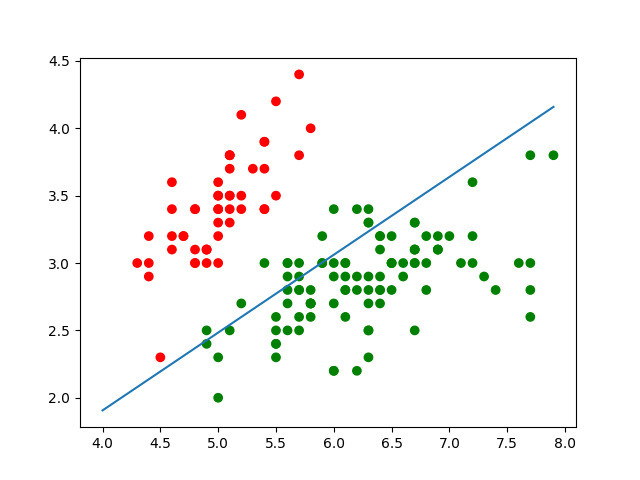像上面的情况基本上循环几十次就稳定在最优解附近。如果学习率等参数没有弄好，可能会出现下面情况：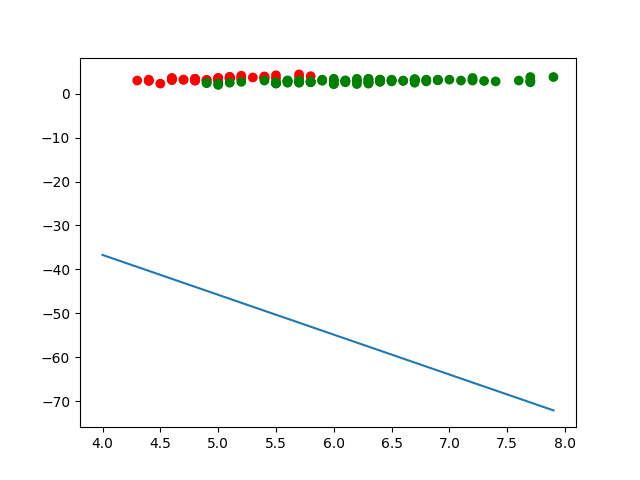## （5）逻辑回归的多分类问题。